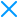April 12, 2017

# Curried Functions in JavaScript

###### By Thomas Greco | 3 min readFunctional programming in JavaScript has become an increasingly sought after skill, and for a good reason. By employing the proper functional programming concepts, users can eliminate problems that come with a global scope, and create applications using same bits of reusable code. Those interested in learning more about FP should check out [Master the JavaScript Interview: What is Functional Programming](https://medium.com/javascript-scene/master-the-javascript-interview-what-is-functional-programming-7f218c68b3a0 "target="_blank) by Eric Elliott, as it does a fantastic job of explaining the topic. Today, we’re going to focus on currying in JavaScript, and take a first-hand look at how we can use curried functions to compose new functions.

#### Why Currying is Useful

When building applications with JavaScript, developers want to ensure their code is written as effectively as possible and follows the D.R.Y. principle of programming (Do Not Repeat Yourself). By using curried functions, our functions are automatically primed for reuse and composition, which plays a huge role in functional programming. To get a better understanding of this, let’s take a look at a non-curried function that simply adds two numbers together.

``````function add2Numbers(a,b) {
return a + b
}
``````

Above, we see a function `add2Numbers` takes the arguments `a` and `b` and adds them together. Below the function, we see the results of logging `add2Numbers()` to the console. In the first instance, our function takes the numbers 4 and 3, and prints out our desired result. Following, we see `add2Numbers()` producing `NaN` (`Not a Number`) because it has not been given a value for `b`. Although the function is completely valid, the way in which it is composed makes it unique to its situation and not very reusable. To better understanding of this, let’s take a look at a curried version of this function and the benefits that it provides users.

``````function curriedAddTwo(a) {
return function (b) {
return a + b;
};
};
``````

The function above works as followed:

1. `curriedAddTwo()` is declared, accepting a single parameter `a`;
2. when called, `curriedAddTwo()` is supplied the argument `1`, returning a new anonymous function which accepts a single parameter `b`;
3. returned function (step 2) is called immediately with argument `2`, returning the expected value `1+2`.

By definition, a curried function is one that takes many parameters and returns a function with a fixed arity of 1. Although that may sound confusing, it simply means that a curried function will always return a function that takes exactly 1 argument. As a result of this, we must pass in arguments in individual parenthesis blocks, or our function will not work.

``````function curriedAddTwo(a) {
return function (b) {
return a + b;
};
};
``````

Additionally, curried functions are also closures, which is a common term in programming. Simply put, a closure is a function bundled with references to its lexical environment. In our case, the inner function is referencing `a` from it’s outer scope, so we know we have a closure. To learn more about closures, [this post](https://medium.com/javascript-scene/master-the-javascript-interview-what-is-a-closure-b2f0d2152b36 "target="_blank) does a fantastic job of explaining them. Continuing on, let’s take a look at how this type of function composition promotes code reuse.

### Re-using Curried functions

In the code block below, we see a new function called `curriedMultiplyTwo()`, which multiplies two numbers together. Taking a look at this example, we see how we can use this function to compose additional functions.

``````function curriedMultiplyTwo(a) {
return function (b) {
return a * b;
};
};
const double = curriedMultiplyTwo(2);
const triple = curriedMultiplyTwo(3);
console.log(double(3); // prints 6
console.log(triple(4); // prints 12
``````

The makeup of `curriedAddTwo()` and `curriedMultiplyTwo()` is almost identical, so we are not going to walk through how our new function works. Instead, we want to focus our attention on the functions created at the bottom of our code block.

``````const double = curriedMultiplyTwo(2);
const triple = curriedMultiplyTwo(3);
``````

The two lines of code above show us just how easy it is to compose functions from a curried function. As their name suggests, `double()` multiplies numbers by 2 and `triple()` multiplies numbers by 3. In both of these functions, Because the first argument from `curriedMultiplyTwo()` is bound within `double()` (`a = 2)` and with `triple()` (`a = 7`), the functions are said to be “closed over”.

### Conclusion

And thus concludes this article on getting started with function currying. Hopefully by now, you have gained an understanding of what a curried function is, and how it works. For many, the concept of currying can seem a bit confusing, so it’s important to understand that it revolves around the ability to reuse functions. That said, those interested in learning more about currying in functional JavaScript should check out this article from SitePoint, [A Beginner's Guide to Currying in JavaScript](https://www.sitepoint.com/currying-in-functional-javascript/ "target="_blank).

Thomas GrecoSoftware engineer focused on building predictable and maintainable software through the use of functional programming, prototypal inheritance, and TDD.

## Subscribe to our weekly newsletter